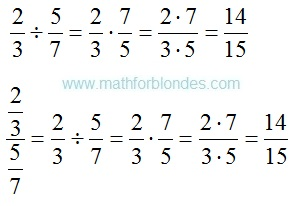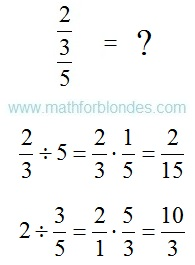## 2.06.2017

### How to divide fraction into fraction?

When performing calculations often there is a question: "How to divide fraction into fraction?". Mathematicians rules tell so:

To divide a number by a fraction, multiply that number by the reciprocal of that fraction.How to divide fraction into fraction?
Transform fraction to division if in numerator and a denominator there are fractions. Be attentive! The numerator and denominator of fraction need to be known. The result depends on it.The numerator and denominator

As fraction to divide into fraction. Formula of division of fractions.Formula of division of fractions
You believe that in mathematician there is a division? The fraction cannot be divided into fraction. They can only be multipled.